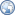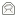## Java RSA Code

A Java implementation of RSA is just a transcription of the algorithm:

```import java.math.BigInteger;
import java.security.SecureRandom;

class Rsa
{
private BigInteger n, d, e;

public Rsa(int bitlen)
{
SecureRandom r = new SecureRandom();
BigInteger p = new BigInteger(bitlen / 2, 100, r);
BigInteger q = new BigInteger(bitlen / 2, 100, r);
n = p.multiply(q);
BigInteger m = (p.subtract(BigInteger.ONE))
.multiply(q.subtract(BigInteger.ONE));
e = new BigInteger("3");
while(m.gcd(e).intValue() > 1) e = e.add(new BigInteger("2"));
d = e.modInverse(m);
}
public BigInteger encrypt(BigInteger message)
{
return message.modPow(e, n);
}
public BigInteger decrypt(BigInteger message)
{
return message.modPow(d, n);
}
}
```

## That's too easy!

Java is a very helpful language for this purpose - it's standard libraries do all the hard work. The main points of java.math.BigInteger are:

• Emulating big numbers using arrays of fixed size integers. Arithmetic operations on big numbers are broken down into sequences of operations on the fixed size numbers, using techniques similar to long multiplication and division used on paper.
• Finding large prime numbers, using the Miller-Rabin probabilistic primality test. This can't prove that numbers are prime; just that it's unlikely they're not prime. The `100` in the `BigInteger` constructors requests 100 primality tests. The probability that the number returned is not prime is less than 1 in 2100.
Apparently an algorithm has now been discovered that can prove primality in polynomial time, so the Java libraries might eventually be updated.
• Calculating modular inverses, using the extended Euclid algorithm.
• Breaking large modular exponentiations into several steps, avoiding the need to store massive intermediate numbers.

Equivalent libraries are available for most programming languages, for example theGMP library for C programmers. You could write one yourself, but these libraries are heavily optimised. They include advanced multiplication/division algorithms and core routines are written in assembler.Harry Callahan has written an alternative implementation which implements the Miller Rabin and Extended Euclid algorithms itself. It also has some good documentation.

## Secure Randomness

For us, java.security.SecureRandom takes care of this, but again this hides a fair bit of hard work. Computers are deterministic, predictable things and not at all suited to generating randomness. The most common source of unpredictability is user input - key strokes and mouse movement. For example, you could time the gap between key strokes, in microseconds, and then take the lowest bit of this number. Techniques like this are random enough for cryptographic purposes. Nowadays, many kernels include this functionality, which is a good idea as they have more time than a process to build up an entropy pool.

## I want to encrypt strings

That code is just illustrative of the core algorithm. If you want to encrypt strings and have the ciphertext as a string, you'll want to use a full-blown encryption package, likeGNU Crypto.

© 1998 - 2012Paul Johnston, distributed under the BSD License   Updated:13 Jul 2009